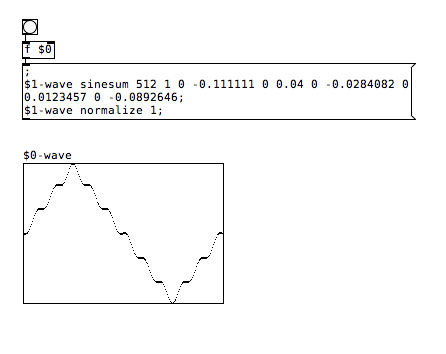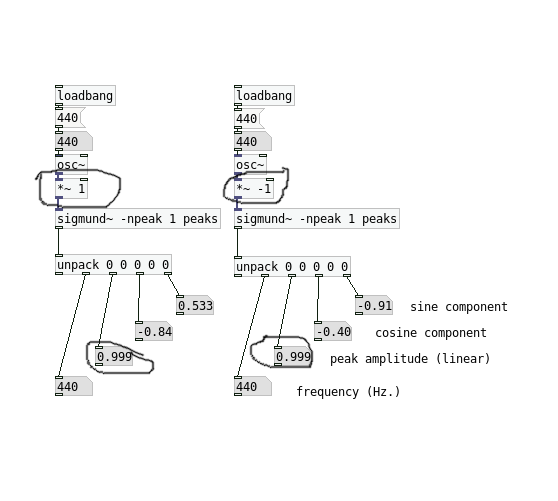• ### fft analysis

Hello !
I need to determine the level of harmonics (for example - 10 harmonics) in a sample (one wave period, for example - one period of a triangular wave length of 512 samples) - how can this be done quickly?

• Posts 7 | Views 3017
• @nionio Hi, you can have a look at [sigmund~].

• @weightless Thanks - this is what I need
)

• Ok.
Triangular wave - is the sum of sinusuids with levels:
1 0 -0.111111 0 0.04 0 -0.0284082 0 0.0123457 0 -0.0892646 ...

here there are negative values. how I can get - calculate - these negative values. I'm just very far from this FFT ...

• @nionio I'm not sure I understand what you are trying to do, but [sigmund~] is for analysing audio, not for generating waveforms.

If you are trying to set a waveform with those values for the partials, you can use the sinesum function like this:just create an array and when you send a bang to the message, the array is resized (in this case to 512 samples) and the waveform is created with the levels for each partial as specified (so first partial is 1, second is 0, third is -0.1111 etc).

• @weightless• the negative amplitudes for the harmonics are relating the phase of that harmonic to the fundamental sinusoid (when it's negative the wave will start out at 1/2 a cycle through)

Posts 7 | Views 3017
Internal error.

Oops! Looks like something went wrong!